Packages

rm(list=ls())
suppressPackageStartupMessages(library(ggplot2))
suppressPackageStartupMessages(library(lmerTest))
suppressPackageStartupMessages(library(lme4))
suppressPackageStartupMessages(library(Matrix))
suppressPackageStartupMessages(library(gridExtra))
suppressPackageStartupMessages(library(plyr))
suppressPackageStartupMessages(library(knitr))
suppressPackageStartupMessages(library(fmsb))
suppressPackageStartupMessages(library(irr))
suppressPackageStartupMessages(library(cowplot))
suppressPackageStartupMessages(library(kableExtra))
suppressPackageStartupMessages(theme_set(theme_bw()))
opts_chunk\$set(fig.width=8, fig.height=5, echo=TRUE, warning=FALSE, message=FALSE, cache=TRUE)

Helper Functions

logodds

This function takes a percentage and returns the log odds.

logodds <- function(p){log(p/(100-p))}

SummarySE

This function can be found on the website “Cookbook for R”.

http://www.cookbook-r.com/Graphs/Plotting_means_and_error_bars_(ggplot2)/#Helper

It summarizes data, giving count, mean, standard deviation, standard error of the mean, and confidence interval (default 95%).

• data: a data frame
• measurevar: the name of a column that contains the variable to be summarized
• groupvars: a vector containing names of columns that contain grouping variables
• na.rm: a boolean that indicates whether to ignore NA’s
• conf.interval: the percent range of the confidence interval (default is 95%)
summarySE <- function(data = NULL, measurevar, groupvars = NULL, na.rm = FALSE, conf.interval = .95, .drop = TRUE) {require(plyr)
# New version of length which can handle NA's: if na.rm==T, don't count them
length2 <- function (x, na.rm = FALSE) {
if (na.rm) sum(!is.na(x))
else       length(x)
}
# This does the summary. For each group's data frame, return a vector with
# N, mean, and sd
datac <- ddply(data, groupvars, .drop = .drop,
.fun = function(xx, col) {
c(N    = length2(xx[[col]], na.rm = na.rm),
mean = mean   (xx[[col]], na.rm = na.rm),
sd   = sd     (xx[[col]], na.rm = na.rm)
)
},
measurevar
)
# Rename the "mean" column
datac <- rename(datac, c("mean" = measurevar))
datac\$se <- datac\$sd / sqrt(datac\$N)  # Calculate standard error of the mean
# Confidence interval multiplier for standard error
# Calculate t-statistic for confidence interval:
# e.g., if conf.interval is .95, use .975 (above/below), and use df=N-1
ciMult <- qt(conf.interval/2 + .5, datac\$N-1)
datac\$ci <- datac\$se * ciMult
return(datac)
}

SummarySEwithin

This function can be found on the website “Cookbook for R”.

http://www.cookbook-r.com/Graphs/Plotting_means_and_error_bars_(ggplot2)/#Helper

From that website:

Summarizes data, handling within-subject variables by removing inter-subject variability. It will still work if there are no within-subject variables. Gives count, un-normed mean, normed mean (with same between-group mean), standard deviation, standard error of the mean, and confidence interval. If there are within-subject variables, it calculates adjusted values using the method from Morey (2008).

• data: a data frame
• measurevar: the name of a column that contains the variable to be summarized
• betweenvars: a vector containing names of columns that are between-subjects variables
• withinvars: a vector containing names of columns that are within-subjects variables
• idvar: the name of a column that identifies each subject (or matched subjects)
• na.rm: a boolean that indicates whether to ignore NA’s
• conf.interval: the percent range of the confidence interval (default is 95%)
summarySEwithin <- function(data = NULL, measurevar, betweenvars = NULL, withinvars = NULL,
idvar = NULL, na.rm = FALSE, conf.interval = .95, .drop = TRUE) {
# Ensure that the betweenvars and withinvars are factors
factorvars <- vapply(data[, c(betweenvars, withinvars), drop = FALSE],
FUN = is.factor, FUN.VALUE = logical(1))
if (!all(factorvars)) {
nonfactorvars <- names(factorvars)[!factorvars]
message("Automatically converting the following non-factors to factors: ",
paste(nonfactorvars, collapse = ", "))
data[nonfactorvars] <- lapply(data[nonfactorvars], factor)
}
# Get the means from the un-normed data
datac <- summarySE(data, measurevar, groupvars = c(betweenvars, withinvars),
na.rm = na.rm, conf.interval = conf.interval, .drop = .drop)
# Drop all the unused columns (these will be calculated with normed data)
datac\$sd <- NULL
datac\$se <- NULL
datac\$ci <- NULL
# Norm each subject's data
ndata <- normDataWithin(data, idvar, measurevar, betweenvars, na.rm, .drop = .drop)
# This is the name of the new column
measurevar_n <- paste(measurevar, "_norm", sep ="")
# Collapse the normed data - now we can treat between and within vars the same
ndatac <- summarySE(ndata, measurevar_n, groupvars = c(betweenvars, withinvars),
na.rm = na.rm, conf.interval = conf.interval, .drop = .drop)
# Apply correction from Morey (2008) to the standard error and confidence interval
# Get the product of the number of conditions of within-S variables
nWithinGroups    <- prod(vapply(ndatac[,withinvars, drop = FALSE], FUN = nlevels,
FUN.VALUE = numeric(1)))
correctionFactor <- sqrt( nWithinGroups / (nWithinGroups-1) )
# Apply the correction factor
ndatac\$sd <- ndatac\$sd * correctionFactor
ndatac\$se <- ndatac\$se * correctionFactor
ndatac\$ci <- ndatac\$ci * correctionFactor
# Combine the un-normed means with the normed results
merge(datac, ndatac)
}

normDataWithin

This function is used by the SummarySEWithin function above. It can be found on the website “Cookbook for R”.

http://www.cookbook-r.com/Graphs/Plotting_means_and_error_bars_(ggplot2)/#Helper

From that website:

Norms the data within specified groups in a data frame; it normalizes each subject (identified by idvar) so that they have the same mean, within each group specified by betweenvars.

• data: a data frame
• idvar: the name of a column that identifies each subject (or matched subjects)
• measurevar: the name of a column that contains the variable to be summarized
• betweenvars: a vector containing names of columns that are between-subjects variables
• na.rm: a boolean that indicates whether to ignore NAs
normDataWithin <- function(data = NULL, idvar, measurevar, betweenvars = NULL,
na.rm = FALSE, .drop = TRUE) {
#library(plyr)
# Measure var on left, idvar + between vars on right of formula.
data.subjMean <- ddply(data, c(idvar, betweenvars), .drop = .drop,
.fun = function(xx, col, na.rm) {
c(subjMean = mean(xx[,col], na.rm = na.rm))
},
measurevar,
na.rm
)
# Put the subject means with original data
data <- merge(data, data.subjMean)
# Get the normalized data in a new column
measureNormedVar <- paste(measurevar, "_norm", sep = "")
data[,measureNormedVar] <- data[,measurevar] - data[,"subjMean"] +
mean(data[,measurevar], na.rm = na.rm)
# Remove this subject mean column
data\$subjMean <- NULL
return(data)
}

myCenter

This function outputs the centered values of a variable, which can be a numeric variable, a factor, or a data frame. It was taken from Florian Jaeger’s blog - https://hlplab.wordpress.com/2009/04/27/centering-several-variables/.

From his blog:

• If the input is a numeric variable, the output is the centered variable

• If the input is a factor, the output is a numeric variable with centered factor level values. That is, the factor’s levels are converted into numerical values in their inherent order (if not specified otherwise, R defaults to alphanumerical order). More specifically, this centers any binary factor so that the value below 0 will be the 1st level of the original factor, and the value above 0 will be the 2nd level.

• If the input is a data frame or matrix, the output is a new matrix of the same dimension and with the centered values and column names that correspond to the colnames() of the input preceded by “c” (e.g. “Variable1” will be “cVariable1”).

myCenter = function(x) {
if (is.numeric(x)) { return(x - mean(x, na.rm = T)) }
if (is.factor(x)) {
x= as.numeric(x)
return(x - mean(x, na.rm = T))
}
if (is.data.frame(x) || is.matrix(x)) {
m= matrix(nrow = nrow(x), ncol = ncol(x))
colnames(m)= paste("c", colnames(x), sep = "")

for (i in 1:ncol(x)) {

m[,i] = myCenter(x[,i])
}
return(as.data.frame(m))
}
}

lizCenter

This function provides a wrapper around myCenter allowing you to center a specific list of variables from a data frame.

• x: data frame
• listfname: a list of the variables to be centered (e.g. list(variable1, variable2))

The output is a copy of the data frame with a column (always a numeric variable) added for each of the centered variables. These columns are labelled with the each column’s previous name, but with “.ct” appended (e.g., “variable1” will become “variable1.ct”).

lizCenter = function(x, listfname)
{
for (i in 1:length(listfname))
{
fname = as.character(listfname[i])
x[paste(fname,".ct", sep="")] = myCenter(x[fname])
}

return(x)
}

lizContrasts

This function can be used to create two centered dummy variables which stand in place of a three-way factor (condition). This allows us to inspect each contrast separately, as well as their interactions with other factors. Other fixed effects in the model can be evaluated as the average effects across all levels of the factor.

The function takes a data frame (d), a factor from that database (condition), which must have three levels, and the name of the level of the factor which is to be used as the baseline for the contrasts (base level).

For example, if d is a data frame with a factor “condition” with three levels “(”lex_skew“,”lex_noskew“, and”mixed“) then lizContrasts(d, d\$condition,”lex_no_skew“) returns a data frame with two (numeric) columns added labelled”lex_noskew_VERSUS_lex_mixed" and “lex_noskew_VERSUS_lex_skew”.

Wherever you would normally use “condition”in a formula in an LME, it can be replaced by (lex_noskew_VERSUS_lex_mixed + “lex_noskew_VERSUS_lex_skew) e.g. ~ (a * condition) becomes ~ (a * (lex_noskew_VERSUS_lex_mixed + lex_noskew_VERSUS_lex_skew)).

lizContrasts = function(d, condition, baselevel)
{

condition = factor(condition)
condition = relevel(condition, baselevel)
a = (contrasts(condition)-apply(contrasts(condition),2,mean))
d\$dummy1[condition == rownames(a)] <- a
d\$dummy1[condition == rownames(a)] <- a
d\$dummy1[condition == rownames(a)] <- a
d\$dummy2[condition == rownames(a)] <- a
d\$dummy2[condition == rownames(a)] <- a
d\$dummy2[condition == rownames(a)] <- a
name1 = paste(baselevel, rownames(a),sep ="_VERSUS_")
name2 = paste(baselevel, rownames(a),sep ="_VERSUS_")
d[name1] = d\$dummy1
d[name2] = d\$dummy2
d\$dummy1 <-NULL
d\$dummy2 <-NULL

return(d)
}

filter2

This is a function which filters a column of data removing values which a certain number of standard deviations above/below the mean for that participant, possibly in some condition/sub-condition.

• im: the input matrix (a data frame)
• svn: a list of the names of factors to be group by (subject name + one or more conditions)
• fn: the name of the column containing the data to be filtered
• lim: how many standard deviations above/below the mean to filter

The function returns an input matrix identical to the original input matrix but with additional columns giving the group means and the “filtered” data

filter2 = function(im, svn, fn, lim)
{
## work out means for each subject for each word
x = list()
y = ""
for(n in svn) x = append(im[n],x)
for(n in svn) y = paste(y,n,sep = "_")
means = aggregate(im[fn], by = x, mean, na.rm = T)
nocols = dim(means)
colnames(means)[nocols] = "means"
sds = aggregate(im[fn], by = x, sd, na.rm = T)
nocols = dim(sds)
colnames(sds)[nocols] = "sds"
gs = merge(means,sds)
## because if there is just one value it does not have a standard deviation and we do not want to just disregard all of these
gs\$sds[is.na(gs\$sds)] = 0
gs\$max = gs\$means + lim*gs\$sds
gs\$min = gs\$means - lim*gs\$sds
im2 = merge(im, gs, sort = F)
im2[paste(fn, "filt", sep = "")] = im2[fn]
cn = dim(im2) ## get colnumber (last one added)
im2[,cn][im2[,fn] > im2\$max] = ""
im2[,cn][im2[,fn] < im2\$min] = ""
im2[,cn] = as.numeric(im2[,cn])
names(im2)[names(im2) == "means"] = paste("mean", y, sep = "_")
names(im2)[names(im2) =="sds"] = paste("sd", y, sep = "_")
names(im2)[names(im2) =="max"] = paste("max", y, sep = "_")
names(im2)[names(im2) =="min"] = paste("min", y, sep = "_")
return(im2)
}

get_coeffs

This function allows us to inspect particular coefficients from the output of an LME model by putting them in table.

• x: the output returned when running lmer or glmer (i.e. an object of type lmerMod or glmerMod)
• list: a list of the names of the coefficients to be extracted (e.g. c(“variable1”, “variable1:variable2”))
get_coeffs <- function(x,list){(as.data.frame(summary(x)\$coefficients)[list,])}

setwd("datafiles")
The working directory was changed to C:/Users/Liz/Desktop/Hanyu_RAnalyses/datafiles inside a notebook chunk. The working directory will be reset when the chunk is finished running. Use the knitr root.dir option in the setup chunk to change the working directory for notebook chunks.
cstc <-read.csv("CSTC-curve.csv", sep = ",", stringsAsFactors = F)
setwd("..")

Individual Aptitude Task 1: Pitch Contour Perception Test (Section 3.2.1)

Figure (Figure 3)

pcpt\$condition = factor(pcpt\$condition, levels = c("0", "1", "2"), labels = c("LV", "HV", "HVB"))
pcpt\$session = factor(pcpt\$session, levels = c("1", "2"), labels = c("Pre-test", "Post-test"))
means = with(pcpt, aggregate(accuracy ~ subject + session + condition, FUN = mean))
x <- summarySEwithin(means, measurevar = "accuracy", betweenvars = c("condition"), withinvars = c("session"), idvar = "subject", na.rm = FALSE, conf.interval = .95)
p1 = ggplot (means, aes(x = condition, y = accuracy, fill = session))
p1 = p1 + geom_violin(colour = "black", scale = "count")
p1 = p1 + scale_fill_manual(name = "Test Session", values = c("Pre-test" = "grey", "Post-test" = "white"), labels = c("Pre-test", "Post-test"))
p1 = p1 + scale_x_discrete(labels = c("LV", "HV", "HVB"))
p1 = p1 + geom_errorbar(aes(ymin = accuracy-ci, ymax = accuracy+ci), width = .4, position = position_dodge(.9), data = x)
p1 = p1 + stat_summary(fun.y = mean, geom = "point", shape = 23, size = 2,position = position_dodge(.9))
p1 = p1 +theme_bw()+ labs(x = "Variability Condition", y = "Mean Accuracy")
print(p1)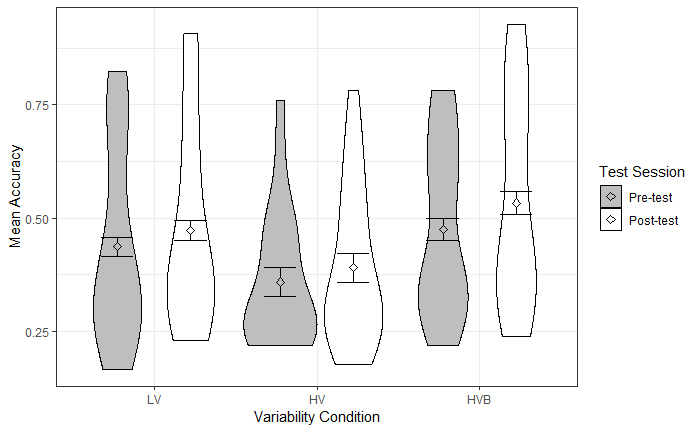Statistical Analysis (Section 3.2.1)

pcpt = lizContrasts(pcpt, pcpt\$condition, "LV")
pcpt_glmer1 = glmer(accuracy ~
session * (LV_VERSUS_HV + LV_VERSUS_HVB)
+ (session|subject),
family = "binomial",
control = glmerControl(optimizer = "bobyqa"),
data = pcpt)
kable (round(summary(pcpt_glmer1)\$coefficients, 6), digits = 3)
Estimate Std. Error z value Pr(>|z|)
(Intercept) -0.324 0.104 -3.098 0.002
sessionPost-test 0.209 0.051 4.134 0.000
LV_VERSUS_HV -0.353 0.255 -1.380 0.168
LV_VERSUS_HVB 0.169 0.256 0.662 0.508
sessionPost-test:LV_VERSUS_HV -0.051 0.124 -0.412 0.680
sessionPost-test:LV_VERSUS_HVB 0.106 0.124 0.853 0.394
datapcpt <- summarySEwithin(means, measurevar = "accuracy", withinvars = c("session"), idvar = "subject", na.rm = FALSE, conf.interval = .95)
kable(datapcpt, digits = 3)
session N accuracy accuracy_norm sd se ci
Post-test 60 0.466 0.466 0.056 0.007 0.014
Pre-test 60 0.424 0.424 0.056 0.007 0.014

Individual Aptitude Task 2: Categorisation of Synthesized Tonal Continua (Section 3.2.2)

We analysed data from the CSTC task adapted from Sadakata and McQueen (2014). We first attempted to follow the procedures in their paper. Specifically, to quantify performance in this task, each subjects’ categorization curve was fitted to a logistic function using the Logistic Curve Fit function in SPSS and a slope coefficient was calculated (Joanisse, Manis, Keating, & Seidenberg, 2000) which was taken to indicate the participants ability to categorize the two tones, with smaller slopes indicating better performance (perfect slope = 0.042). Sadakata and McQueen (2014), report that they removed any participant with a slope measure greater than 1.2 from the analysis, suggesting that slopes above this threshold were considered to be poorly fit. However following this process with our participants, the majority were above this threshold (43 out of 60). Given this, we attempted an alternative method of deriving slope coefficients for each participant using a logistic mixed effect model (Schultz, Llanos, & Francis, 2003) with the predicted variable being which of two tones the participants chose on each trial and the predictor being the tone step presented on each trial (varying from 1-6 where 1 is most like Tone 2, and 6 is most like Tone 3). Random intercepts and slopes for tone step were fit by participant and the individual slope coefficients for each participant were extracted from the by-participant random slopes fit in the model. Because the random slopes represent adjustments to the fixed effect slope, more positive slopes represent sharper categorization responses, i.e. more sensitivity to differences in tone step, while more negative slopes represent flatter categorization responses, or in extreme cases reversed responses, and slopes close to 0 reflect responses close to the mean. These slopes could thus be taken to be indicators of individual differences. When we compared these slopes to the ones derived from SPSS we found that they were very similar. The same participants who failed the criteria of Sadakata and McQueen also had very shallow slopes using the logistic regression method. The analyses conducted in this script were done with the individual difference measure extracted from the logistic regression.

This figure shows the individual curves for each group. It also shows the raw data that the slopes are based on.

cstc\$session = factor(cstc\$session, levels = c("1","2"), labels = c("Pre-test", "Post-test"))
cstc\$condition = factor(cstc\$condition, levels = c("0", "1", "2"), labels = c("LV", "HV", "HVB"))
# Two participants showed the completely reversed pattern. Here we reverse the coding of these 2 participants
cstc\$category[cstc\$subject == "5"|cstc\$subject == "36"] <- abs(cstc\$category[cstc\$subject == "5"|cstc\$subject == "36"] - 1)
ggplot(cstc, aes(x = step, y = category)) +
stat_smooth(aes(group = subject), method = "glm", method.args = list(family = "binomial"), size = 0.5, se = FALSE, show.legend = FALSE, color = "#cce2ef") + # individual regression lines
stat_smooth(method = "glm", method.args = list(family = "binomial"), size = 2, se = FALSE, show.legend = FALSE, color = "#B01326") + # group mean regression line
ylim(0, 1) + scale_x_continuous(breaks = 1:7) + facet_grid(~condition) +
xlab("tone step") + ylab("Proportion Tone X responses") + theme_bw() +
theme(plot.title = element_text(hjust = 0.5, face = "bold"))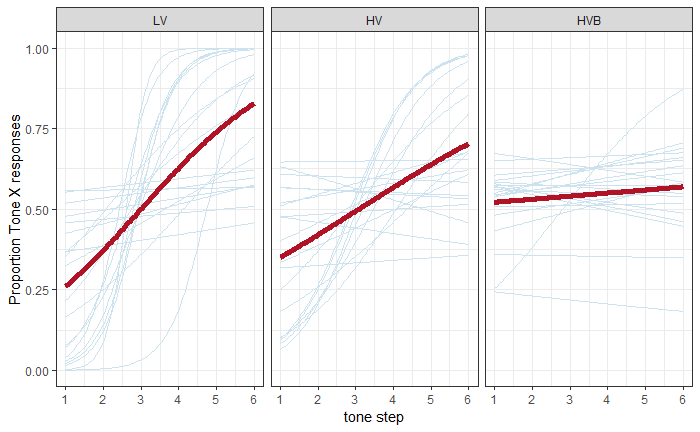Extract slope coffcient for each participant and descriptives

cstc\$session <- as.numeric(cstc\$session)
cstc\$session.std <- scale(cstc\$session)
cstc\$step.std <- scale(cstc\$step)
f1 <-  factor(c("HV", "LV"))
df1 <- data.frame(f1, subject = 1:40)
f1 <-  factor("HVB")
df2 <- data.frame(f1, subject = 41:60)
df3 <- rbind.fill(df1, df2)
lmfits_pre <- glmer(category ~ step.std + (step.std|subject), data = cstc[cstc\$session == 1,], family = "binomial", control = glmerControl(optimizer = "bobyqa"))
pre = ranef(lmfits_pre)\$subject
pre = cbind (pre, df3)
pre\$session = "Pre-test"
lmfits_post <- glmer(category ~ step.std + (step.std|subject), data = cstc[cstc\$session == 2,], family = "binomial", control = glmerControl(optimizer = "bobyqa"))
post = ranef(lmfits_post)\$subject
post = cbind (post, df3)
post\$session = "Post-test"
cstc2 <- rbind(pre,post)
names(cstc2)[names(cstc2) == "step.std"] <- "slope"
names(cstc2)[names(cstc2) == "(Intercept)"] <- "intercept"
names(cstc2)[names(cstc2) == "f1"] <- "condition"
# reorder the levels so that they match previous data set
cstc2\$condition = factor (cstc2\$condition, levels =  c("LV","HV","HVB") )
cstc2\$session = factor (cstc2\$session, levels =  c("Pre-test","Post-test") )
means = with(cstc2, aggregate(slope ~ subject + session + condition, FUN = mean))
x <- summarySEwithin(cstc2, measurevar = "slope", betweenvars = c("condition"), withinvars = c("session"), idvar = "subject", na.rm = FALSE, conf.interval = .95)
kable(x, digits = 2)
condition session N slope slope_norm sd se ci
HV Post-test 20 -0.17 -0.12 0.60 0.13 0.28
HV Pre-test 20 0.02 0.07 0.60 0.13 0.28
HVB Post-test 20 -0.77 -0.15 0.58 0.13 0.27
HVB Pre-test 20 -0.53 0.10 0.58 0.13 0.27
LV Post-test 20 0.86 0.19 0.46 0.10 0.21
LV Pre-test 20 0.44 -0.24 0.46 0.10 0.21

Graph

p2 = ggplot (means, aes(x = condition, y = slope, fill = session))
p2 = p2 + geom_violin(colour = "black", scale = "count")
p2 = p2 + scale_fill_manual(name = "Test session", values = c("Pre-test" = "grey", "Post-test" = "white"))
p2 = p2 + scale_x_discrete(labels = c("Low Variability", "High Variability", "High Varaibility Blocking"))
p2 = p2 + geom_errorbar(aes(ymin = slope-ci, ymax = slope+ci), width = .4, position = position_dodge(.9), data = x)
p2 = p2 + stat_summary(fun.y = mean, geom = "point", shape = 23, size = 2, position = position_dodge(.9))
p2 = p2 + theme_bw()
print(p2)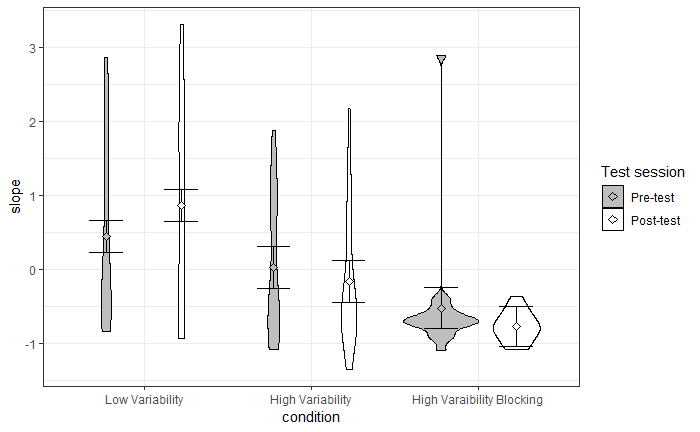rm(means)
rm(x)

Statistical Analysis

cstc2 = lizContrasts(cstc2, cstc2\$condition, "LV")
cstc_lm = lm(slope ~ session * (LV_VERSUS_HV + LV_VERSUS_HVB) , data = cstc2)

Look at differences between conditions at pre-test:

round (get_coeffs(cstc_lm,c("LV_VERSUS_HV","LV_VERSUS_HVB")),3)

Look at the relationship between PCPT and CSTC and set up ID_pre table

ID_pre = merge(

with(subset(pcpt, session %in% "Pre-test"), aggregate(accuracy ~ subject + session + condition, FUN = mean)),

with(subset(cstc2, session %in% "Pre-test"), aggregate(slope ~ subject + session + condition, FUN = mean)), by = "subject")
ID_pre\$aptitude_pcpt = ID_pre\$accuracy *10
ID_pre\$session = NULL
cor.test(ID_pre\$slope, ID_pre\$accuracy)

Pearson's product-moment correlation

data:  ID_pre\$slope and ID_pre\$accuracy
t = 2.9688, df = 58, p-value = 0.004341
alternative hypothesis: true correlation is not equal to 0
95 percent confidence interval:
0.1203742 0.5650138
sample estimates:
cor
0.3631974

Correlation Figure

ggplot(ID_pre, aes(x = accuracy, y = slope, color = condition.x)) +
geom_point(size = 5)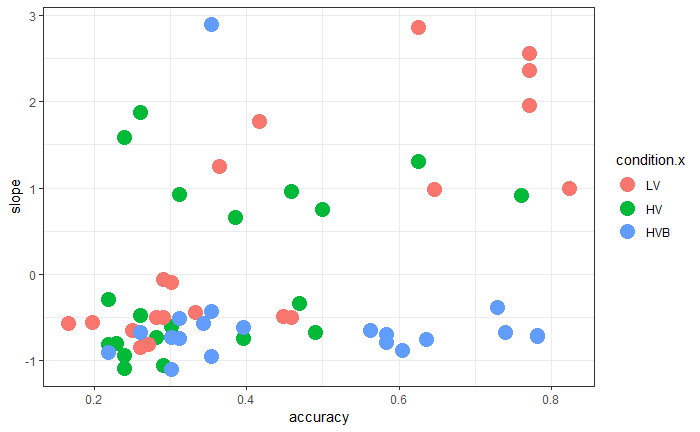Create a median split (this is used later in plotting, but not in analyses)

ID_pre\$aptitude2 = 1
ID_pre\$aptitude2[ID_pre\$accuracy < median(ID_pre\$accuracy)] = 0
ID_pre\$aptitude3 = 1
ID_pre\$aptitude3[ID_pre\$slope < median(ID_pre\$slope)] = 0
ID_pre\$aptitude2 = factor(ID_pre\$aptitude2, levels = c("1", "0"), labels = c ("HA", "LA"))
ID_pre\$aptitude3 = factor(ID_pre\$aptitude3, levels = c("0", "1"), labels = c ("HA", "LA"))

Production Reliability Analysis (Section 3.5.1.1)

Checking the accuracy of ratings of production data by native speakers

#Remove participant 48
PRO = PRO[!(PRO\$subject=="48"),]
PRO\$session = factor(PRO\$session, levels = c("pretest", "posttest", "nativespeaker", "picturenaming"), labels = c("Pre-test", "Post-test","Native-Speaker", "Picture-Naming"))
PC = subset(PRO, session %in% "Native-Speaker")
means = mean(PC\$tone_score)
means2 = mean(PC\$pinyin_score)
means3 = mean(PC\$rating)
means4 = mean(PC\$tone_score_rater1)
means5 = mean(PC\$pinyin_score_rater1)
means6 = mean(PC\$rating_rater1)
Rater2 = c(means, means2, means3)
Rater1 = c(means4, means5, means6)
Task = factor (c("Tone accuracy","Pinyin accuracy","Tone rating"))
Table = data.frame ("Task" = Task, "Rater 1" = Rater1, "Rater 2" = Rater2)
Table
rm(means, means2, means3, means4, means5, means6, Rater2, Rater1, Task)

Checking the correlation between Rater1 and Rater2

These correlations are reported for ratings of the experimental data

CWR = subset(PRO, session %in% c("Pre-test", "Post-test"))
CPN = subset(PRO, session %in% "Picture-Naming")
cor1 = Kappa.test(CWR\$tone_score, CWR\$tone_score_rater1, conf.level = 0.95)
cor2 = Kappa.test(CWR\$pinyin_score, CWR\$pinyin_score_rater1, conf.level = 0.95)
cor3 = icc(cbind(CWR\$rating, CWR\$rating_rater1), "twoway", "consistency")
cor4 = Kappa.test(CPN\$tone_score, CPN\$tone_score_rater1, conf.level = 0.95)
cor5 = Kappa.test(CPN\$pinyin_score, CPN\$pinyin_score_rater1, conf.level = 0.95)
cor6 = icc(cbind(CPN\$rating, CPN\$rating_rater1), "twoway", "consistency")

Rater accuracy of tone identification in Word Repetition

cor1
\$Result

Estimate Cohen's kappa statistics and test the null hypothesis that the extent of agreement is same as random (kappa=0)

data:  CWR\$tone_score and CWR\$tone_score_rater1
Z = 25.477, p-value < 2.2e-16
95 percent confidence interval:
0.3682339 0.4197987
sample estimates:
 0.3940163

\$Judgement
 "Fair agreement"

Rater accuracy of pinyin identification in Word Repetition

cor2
\$Result

Estimate Cohen's kappa statistics and test the null hypothesis that the extent of agreement is same as random (kappa=0)

data:  CWR\$pinyin_score and CWR\$pinyin_score_rater1
Z = 28.691, p-value < 2.2e-16
95 percent confidence interval:
0.3105457 0.3526499
sample estimates:
 0.3315978

\$Judgement
 "Fair agreement"

Rater accuracy of tone rating in Word Repetition

cor3
Single Score Intraclass Correlation

Model: twoway
Type : consistency

Subjects = 8496
Raters = 2
ICC(C,1) = 0.22

F-Test, H0: r0 = 0 ; H1: r0 > 0
F(8495,8495) = 1.56 , p = 5.07e-94

95%-Confidence Interval for ICC Population Values:
0.2 < ICC < 0.24

Rater accuracy of tone identification in Picture Naming

cor4
\$Result

Estimate Cohen's kappa statistics and test the null hypothesis that the extent of agreement is same as random (kappa=0)

data:  CPN\$tone_score and CPN\$tone_score_rater1
Z = 31.149, p-value < 2.2e-16
95 percent confidence interval:
0.6408330 0.7035999
sample estimates:
 0.6722164

\$Judgement
 "Substantial agreement"

Rater accuracy of pinyin identification in Picture Naming

cor5
\$Result

Estimate Cohen's kappa statistics and test the null hypothesis that the extent of agreement is same as random (kappa=0)

data:  CPN\$pinyin_score and CPN\$pinyin_score_rater1
Z = 25.434, p-value < 2.2e-16
95 percent confidence interval:
0.4969453 0.5672871
sample estimates:
 0.5321162

\$Judgement
 "Moderate agreement"

Rater accuracy of tone rating in Picture Naming

cor6
Single Score Intraclass Correlation

Model: twoway
Type : consistency

Subjects = 2124
Raters = 2
ICC(C,1) = 0.372

F-Test, H0: r0 = 0 ; H1: r0 > 0
F(2123,2123) = 2.18 , p = 5.14e-71

95%-Confidence Interval for ICC Population Values:
0.335 < ICC < 0.408

Word Repetition (Section 3.5.1)

Word Repetition - Tone accuracy (Section 3.5.1.2)

Label Parameters and remove unidentifiable trials

NWR = CWR[CWR\$tone != 0, ]
NWR\$condition = factor(NWR\$condition, levels = c("0", "1","2"), labels = c("LV", "HV", "HVB"))
NWR\$wordtype = factor(NWR\$wordtype, levels = c("trained", "untrained"), labels = c("Trained Item", "Untrained Item"))
NWR2 = merge(NWR, ID_pre)

Figures

means = with(NWR2, aggregate(tone_score ~ subject + session + condition + wordtype, FUN = mean))
meansb = with(NWR2, aggregate(tone_score ~ subject + session + condition + wordtype + aptitude2, FUN = mean))
means2b = with(NWR2, aggregate(tone_score ~ subject + session + condition + wordtype + aptitude3, FUN = mean))
xb <- summarySEwithin(means, measurevar = "tone_score", betweenvars = c("condition"), withinvars = c("wordtype", "session"), idvar = "subject", na.rm = FALSE, conf.interval = .95)
graphdatab <- summarySEwithin(meansb, measurevar = "tone_score", betweenvars = c("condition", "aptitude2"), withinvars = c("session", "wordtype"), idvar = "subject", na.rm = FALSE, conf.interval = .95)
graphdata2b <- summarySEwithin(means2b, measurevar = "tone_score", betweenvars = c("condition", "aptitude3"), withinvars = c("session", "wordtype"), idvar = "subject", na.rm = FALSE, conf.interval = .95)

Main Graph (Figure 7)

WRp1 = ggplot (means, aes(x = condition, y = tone_score, fill = session))
WRp1 = WRp1 + facet_wrap(~wordtype, ncol = 2)
WRp1 = WRp1 + geom_violin(colour = "black", scale = "count")
WRp1 = WRp1 + scale_fill_manual(name = "Test Session", values = c("Pre-test" = "grey", "Post-test" = "white"), labels = c("Pre-test", "Post-test"))
WRp1 = WRp1 + scale_x_discrete(labels = c("LV", "HV", "HVB"))
WRp1 = WRp1 + geom_errorbar(aes(ymin = tone_score-ci, ymax = tone_score+ci), width = .4, position = position_dodge(.9), data = xb)
WRp1 = WRp1 + stat_summary(fun.y = mean, geom = "point", shape = 23, size = 2,position = position_dodge(.9))
WRp1 = WRp1 +theme_bw()+ ggtitle('Word Repetition: Tone accuracy')
WRp1 = WRp1 + labs(x = "Variability Condition", y = "Mean Accuracy")
print(WRp1)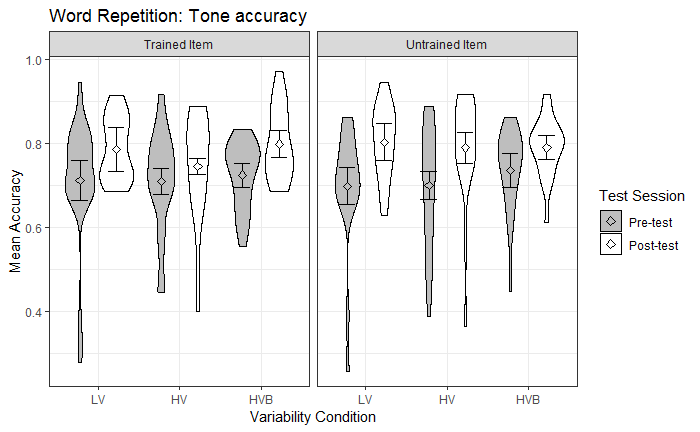Graph (Figure 12, Left panel)

WRp2 = ggplot (meansb, aes(x = condition, y = tone_score, fill = session))
WRp2 = WRp2 + facet_wrap(~wordtype + aptitude2, ncol = 2)
WRp2 = WRp2 + geom_violin(colour = "black", scale = "count")
WRp2 = WRp2 + scale_fill_manual(name = "Test Session", values = c("Pre-test" = "grey", "Post-test" = "white"), labels = c("Pre-test", "Post-test"))
WRp2 = WRp2 + scale_x_discrete(labels = c("LV", "HV", "HVB"))
WRp2 = WRp2 + geom_errorbar(aes(ymin = tone_score-ci, ymax = tone_score+ci), width = .4, position = position_dodge(.9), data = graphdatab)
WRp2 = WRp2 + stat_summary(fun.y = mean, geom = "point", shape = 23, size = 2,position = position_dodge(.9))
WRp2 = WRp2 + theme_bw()+ ggtitle('Word Repetition: Tone accuracy')
WRp2 = WRp2 + labs(x = "Variability Condition", y = "Mean Accuracy")
WRp2.m = WRp2 + theme(legend.position = "NULL")
print(WRp2)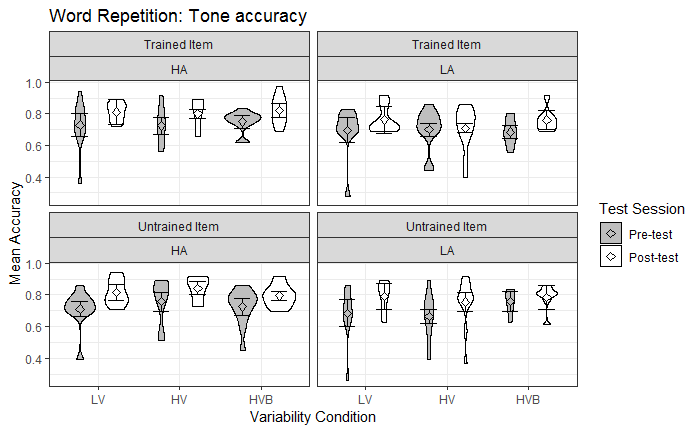CSTC Graph (Not used)

WRp3 = ggplot (means2b, aes(x = condition, y = tone_score, fill = session))
WRp3 = WRp3 + facet_wrap(~wordtype + aptitude3, ncol = 2)
WRp3 = WRp3 + geom_violin(colour = "black", scale = "count")
WRp3 = WRp3 + scale_fill_manual(name = "Test Session", values = c("Pre-test" = "grey", "Post-test" = "white"), labels = c("Pre-test", "Post-test"))
WRp3 = WRp3 + scale_x_discrete(labels = c("LV", "HV", "HVB"))
WRp3 = WRp3 + geom_errorbar(aes(ymin = tone_score-ci, ymax = tone_score+ci), width = .4, position = position_dodge(.9), data = graphdata2b)
WRp3 = WRp3 + stat_summary(fun.y = mean, geom = "point", shape = 23, size = 2,position = position_dodge(.9))
WRp3 = WRp3 + theme_bw()+ ggtitle('Word Repetition: Tone accuracy')
WRp3 = WRp3 + labs(x = "Variability Condition", y = "Mean Accuracy")
print(WRp3)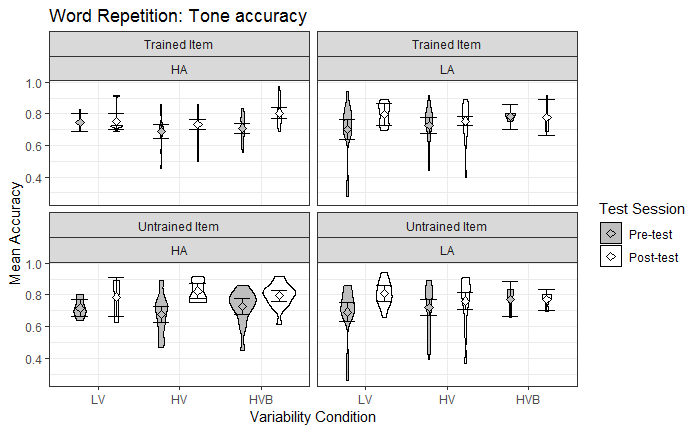Statistical Analysis

Main Model (without aptitude predictors; Section 3.5.1.2)

NWR2 = lizCenter(NWR2, list("wordtype", "condition"))
NWR2 = lizContrasts(NWR2, NWR2\$condition, "LV")
nw_glmer = glmer(tone_score ~ wordtype.ct * (LV_VERSUS_HV + LV_VERSUS_HVB) * session
+ (wordtype.ct * session||subject),
family = "binomial",
control = glmerControl(optimizer = "bobyqa"),
data = NWR2)
singular fit

Analysis with PCPT accuracy (Section 3.7)

NWR2 = lizCenter(NWR2, list("session", "aptitude_pcpt", "slope", "wordtype"))
nw_glmer_pcpt = glmer(tone_score ~ wordtype.ct * (LV_VERSUS_HV + LV_VERSUS_HVB) * session.ct
+ aptitude_pcpt.ct
+ aptitude_pcpt.ct : session.ct
+ aptitude_pcpt.ct : (LV_VERSUS_HV + LV_VERSUS_HVB) : session.ct
+ aptitude_pcpt.ct : (LV_VERSUS_HV + LV_VERSUS_HVB) : session.ct : wordtype.ct
+ (wordtype.ct * session.ct||subject)
, family="binomial" ,
control = glmerControl(optimizer = "bobyqa"),
data = NWR2)
singular fit
Check that we get the same pattern of results for the experimentally manipulated variables as in the previous model
kable(get_coeffs(nw_glmer_pcpt, c("session.ct",
"wordtype.ct:session.ct",
"LV_VERSUS_HV:session.ct",
"LV_VERSUS_HVB:session.ct",
"wordtype.ct:LV_VERSUS_HV:session.ct",
"wordtype.ct:LV_VERSUS_HVB:session.ct" )), digits = 3)
Estimate Std. Error z value Pr(>|z|)
session.ct 0.401 0.077 5.186 0.000
wordtype.ct:session.ct 0.089 0.109 0.816 0.415
LV_VERSUS_HV:session.ct -0.089 0.189 -0.470 0.638
LV_VERSUS_HVB:session.ct -0.155 0.184 -0.843 0.399
wordtype.ct:LV_VERSUS_HV:session.ct 0.076 0.261 0.289 0.772
wordtype.ct:LV_VERSUS_HVB:session.ct -0.340 0.258 -1.319 0.187

Analysis with CSTC slope (Section 3.7)

#Model did not converge so remove slope.ct : (LV_VERSUS_HV + LV_VERSUS_HVB) : session.ct : wordtype.ct
nw_glmer_cstc = glmer(tone_score ~ wordtype.ct * (LV_VERSUS_HV + LV_VERSUS_HVB) * session.ct
+ slope.ct
+ slope.ct : session.ct
+ slope.ct : (LV_VERSUS_HV + LV_VERSUS_HVB) : session.ct
+ (wordtype.ct * session.ct|subject)
, family = "binomial" ,
control = glmerControl(optimizer = "bobyqa", optCtrl=list(maxfun=1e6)),
data = NWR2)
singular fit
Check that get the same pattern of results for experimentally manipulated variables as in previous model
kable(get_coeffs(nw_glmer_cstc, c("session.ct", "wordtype.ct:session.ct", "wordtype.ct:LV_VERSUS_HV:session.ct", "wordtype.ct:LV_VERSUS_HVB:session.ct")), digits = 3)
Estimate Std. Error z value Pr(>|z|)
session.ct 0.336 0.078 4.327 0.000
wordtype.ct:session.ct 0.119 0.106 1.123 0.262
wordtype.ct:LV_VERSUS_HV:session.ct 0.147 0.254 0.580 0.562
wordtype.ct:LV_VERSUS_HVB:session.ct -0.307 0.257 -1.194 0.233

Word Repetition - Pinyin Accuracy (Section 3.5.1.3)

Figures

means = with(NWR2, aggregate(pinyin_score ~ subject + session + condition + wordtype, FUN = mean))
meansb = with(NWR2, aggregate(pinyin_score ~ subject + session + condition + wordtype + aptitude2, FUN = mean))
means2b = with(NWR2, aggregate(pinyin_score ~ subject + session + condition + wordtype + aptitude3, FUN = mean))
xb <- summarySEwithin(means, measurevar = "pinyin_score", betweenvars = c("condition"), withinvars = c("wordtype", "session"), idvar = "subject", na.rm = FALSE, conf.interval = .95)
graphdatab <- summarySEwithin(meansb, measurevar = "pinyin_score", betweenvars = c("condition", "aptitude2"), withinvars = c("session", "wordtype"), idvar = "subject", na.rm = FALSE, conf.interval = .95)
graphdata2b <- summarySEwithin(means2b, measurevar = "pinyin_score", betweenvars = c("condition", "aptitude3"), withinvars = c("session", "wordtype"), idvar = "subject", na.rm = FALSE, conf.interval = .95)

Main Graph (Figure 8)

WRp9 = ggplot (means, aes(x = condition, y = pinyin_score, fill = session))
WRp9 = WRp9 + facet_wrap(~wordtype, ncol = 2)
WRp9 = WRp9 + geom_violin(colour = "black", scale = "count")
WRp9 = WRp9 + scale_fill_manual(name = "Test Session", values = c("Pre-test" = "grey", "Post-test" = "white"), labels = c("Pre-test", "Post-test"))
WRp9 = WRp9 + scale_x_discrete(labels = c("LV", "HV", "HVB"))
WRp9 = WRp9 + geom_errorbar(aes(ymin = pinyin_score-ci, ymax = pinyin_score+ci), width = .4, position = position_dodge(.9), data = xb)
WRp9 = WRp9 + stat_summary(fun.y = mean, geom = "point", shape = 23, size = 2, position = position_dodge(.9))
WRp9 = WRp9 + theme_bw() + ggtitle('Word Repetition: Pinyin accuracy')
WRp9 = WRp9 + labs(x = "Variability Condition", y = "Mean Accuracy")
print(WRp9)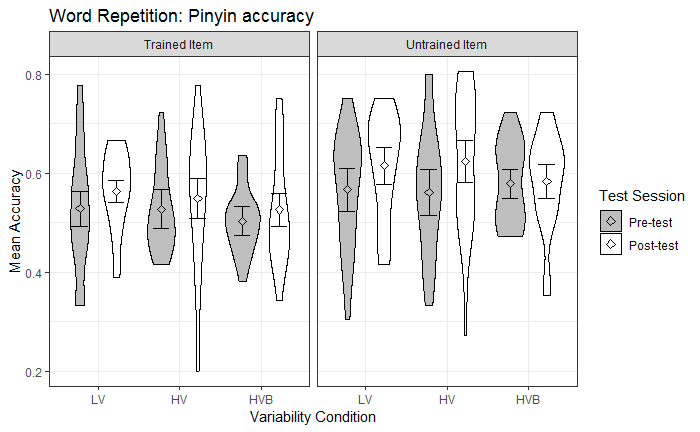PCPT Graph (Figure 12, Right panel)

WRp10 = ggplot (meansb, aes(x = condition, y = pinyin_score, fill = session))
WRp10 = WRp10 + facet_wrap(~wordtype + aptitude2, ncol = 2)
WRp10 = WRp10 + geom_violin(colour = "black", scale = "count")
WRp10 = WRp10 + scale_fill_manual(name = "Test Session", values = c("Pre-test" = "grey", "Post-test" = "white"), labels = c("Pre-test", "Post-test"))
WRp10 = WRp10 + scale_x_discrete(labels = c("LV", "HV", "HVB"))
WRp10 = WRp10 + geom_errorbar(aes(ymin = pinyin_score-ci, ymax = pinyin_score+ci), width = .4, position = position_dodge(.9), data = graphdatab)
WRp10 = WRp10 + stat_summary(fun.y = mean, geom = "point", shape = 23, size = 2,position = position_dodge(.9))
WRp10 = WRp10 + theme_bw()+ ggtitle('Word Repetition: Pinyin accuracy')
WRp10 = WRp10 + labs(x = "Variability Condition", y = "Mean Accuracy")
print(WRp10)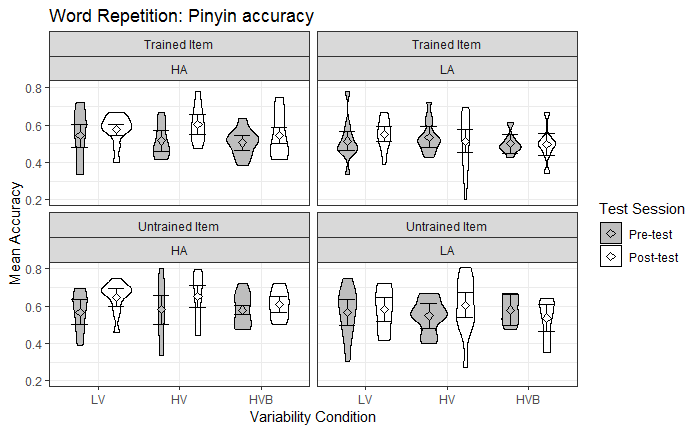CSTC Graph (Not used)

WRp11 = ggplot (means2b, aes(x = condition, y = pinyin_score, fill = session))
WRp11 = WRp11 + facet_wrap(~wordtype + aptitude3, ncol = 2)
WRp11 = WRp11 + geom_violin(colour = "black", scale = "count")
WRp11 = WRp11 + scale_fill_manual(name = "Test Session", values = c("Pre-test" = "grey", "Post-test" = "white"), labels = c("Pre-test", "Post-test"))
WRp11 = WRp11 + scale_x_discrete(labels = c("LV", "HV", "HVB"))
WRp11 = WRp11 + geom_errorbar(aes(ymin = pinyin_score-ci, ymax = pinyin_score+ci), width = .4, position = position_dodge(.9), data = graphdata2b)
WRp11 = WRp11 + stat_summary(fun.y = mean, geom = "point", shape = 23, size = 2,position = position_dodge(.9))
WRp11 = WRp11 + theme_bw()+ ggtitle('Word Repetition: Pinyin accuracy')
WRp11 = WRp11 + labs(x = "Variability Condition", y = "Mean Accuracy")
print(WRp11)